# How do I know if my language model is gender-biased?

Some examples of bias measures for language models.

The language model (LM) is an essential building block of current AI systems dealing with natural language, and has proven to be useful in tasks as diverse as sentiment analysis, text generation, translations, and summarizing. These LMs are typically based on deep neural networks and trained on vast amounts of training data, which makes these so effective, but this doesn’t come without any problems.

LMs have been shown to learn undesirable biases towards certain social groups, which may unfairly influence the decisions, recommendations or texts that AI systems building on those LMs generate. However, the black-box nature of deep neural networks and the fact that these are trained on very large datasets makes it difficult to understand how LMs are biased. Nevertheless, researchers have proposed many different techniques to study the biases that are present in current natural language technologies.

In this blog post, we will give some examples of techniques we use in our lab to measure one well-studied type of bias, gender bias, in the different representations of language models.

## What are language models?

A language model (LM) is a statistical model to predict the next likely word given a sequence of other words. The most successful ones are based on deep neural networks. There exist many different types of networks (e.g. LSTM, Transformer), but all rely on encoding words or word parts as vector representations and passing these through the “hidden layers” of the network resulting in “contextual embeddings” (called such because contextual information is represented in the vectors, such as information on the neighbouring words). Typically, LMs have billions of parameters that are trained on huge datasets, which makes these so effective but also very complex systems.

While we have a lot of examples showing that LMs exhibit undesirable gender biases, it remains challenging to measure gender bias in these models. In this post, we will focus on three stages in a typical LM pipeline, as highlighted in the figure below, and give examples of how you can measure gender bias in these different representations.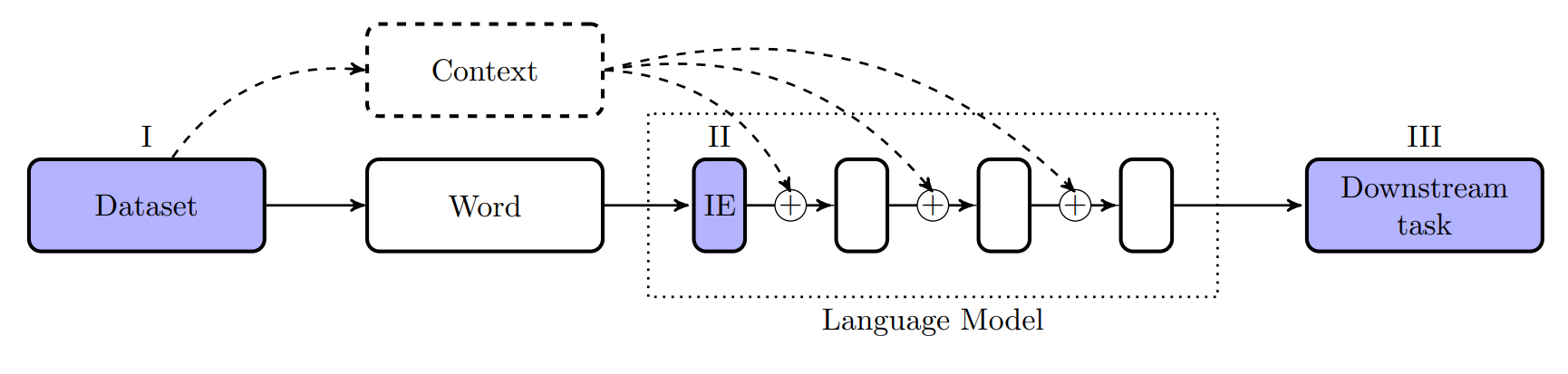First, we discuss how gender bias can be measured in the training dataset of a model (I) and at the end of the pipeline in a downstream task that makes use of the contextual embeddings of the model (III). We then give an example of how to study gender bias in the internal state of the LM, by studying the input embeddings (IE), wich make up the first layer of the LM (II). However, before we discuss these methods, we give a brief explanation of what we mean by gender bias.

## Gender bias of occupation terms

Defining (undesirable) bias is a complicated matter, and there are many definitions and frameworks discussed in the literature [e.g. 1, 2, 3]. In this post, we use gender-neutrality as the norm, and define bias as any significant deviation from a 50-50% distribution in preferences, probabilities or similarities.

Inspired by previous work [4, 5, 6], we consider the gender bias of occupation terms in the examples. To quantify the gender bias of an occupation, we often use unambiguously gendered word-pairs (e.g. “man”-“woman”, “he”-“she”, “king”-“queen”) in our measures. It is important to keep in mind, however, that ‘gender’ is a multifaceted concept, which is much more complicated than a simple male-female dichotomy suggests [7, 8].

## Dataset bias

It is not a strange idea to investigate the gender bias in the training data of the LM. In the end, the model learns these biases from the data in some way or another. A typical approach to quantify the dataset bias, is to use measurable features in the dataset, such as word counts or how often gendered words co-occur with the words of interest [10, 11, 12, 13]. While these statistics can give some indication of possible sources of bias, it doesn’t tell use about more nuanced and implicit ways that gender bias can be present in texts that may still be picked up by LMs. Other researchers use special trained classifiers for showing gender bias in texts [14, 15, 16]. However, how these biases are learnt from texts by LMs and what features are important is still an active area of research.

## Downstream bias

Most work on the bias of LMs is focused on the output. Any bias in the predictions, recommendations, and texts generated in the downstream task has the potential to actually harm people by unfair behaviour . This bias is typically tested by challenge sets: carefully constructed sets of sentences used to probe the model for any specific biases.

One example of a challenge set is the semantic textual similarity task for bias (STS-B) . In this task, the LM is used for estimating the similarity of three sentences, containing either the word “man”, “woman”, or an occupation term. Then the gender bias for that occupation term is the difference in the similarity averaged over a set of template sentences. Below you see an example for finding the gender bias for “janitor” using one template sentence, where the final score is 0.75 - 0.54 = 0.21 (male bias).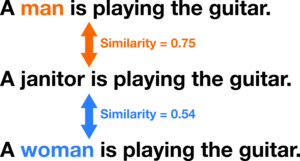## Embedding bias

We have seen some examples of measuring gender bias in the training data of an LM and at its output in a downstream task. Something that is not studied as much, is the gender bias in the internal states of an LM. Because of the black-box nature of deep neural networks this is notoriously difficult.

However, there is one layer in a typical LM that is actually very suitable for measuring bias: the input embeddings. The input embeddings are the first layer of the LM and encode word (parts) into word vectors, which can be used in the other layers. The input embeddings actually have a similar representation to static word embeddings, for which researchers have actually developed many techniques to measure gender bias. One class of bias measures relies on finding a linear gender subspace. Let us explain one of these methods from Ravfogel et al. .

For this measure, we start with a set of unambiguously female and male words (e.g. “man”-“woman”, “he”-“she”, “son”-“daughter”) and train a linear classifier, such as a support vector machine (SVM), to predict the gender of each word.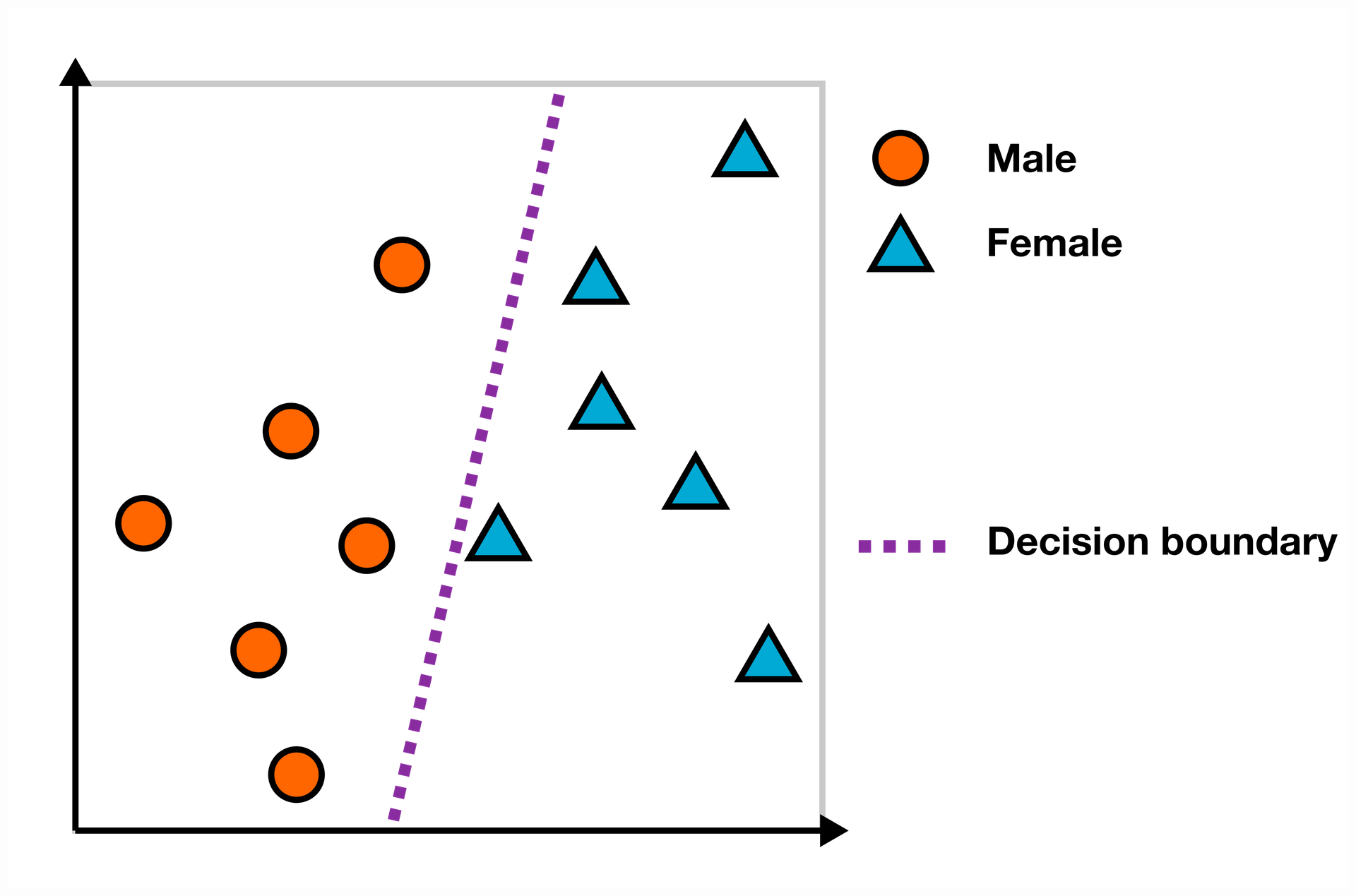We then define the gender subspace as the axis orthogonal to the decision boundary of the linear SVM that is trained to predict the gender for a set of male and female words.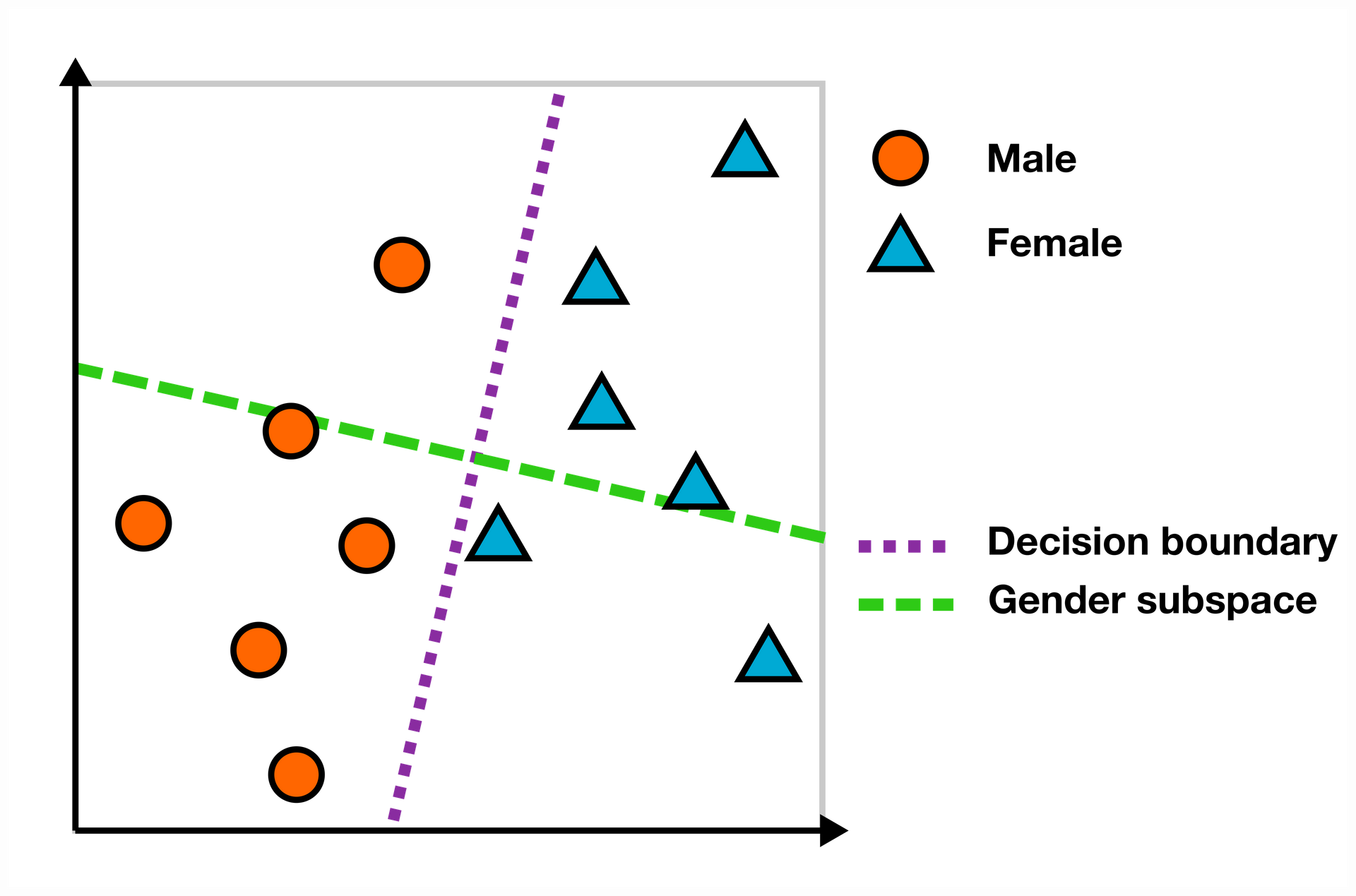Now we can use the gender subspace for quantifying the gender bias of, for example, occupation terms. For finding the bias score of a word in the embedding space, we use the scalar projection on the gender subspace. In the example below, words like “engineer” and “scientist” are on the “male” side of the subspace (to the left of the purple decision boundary), while “nurse” and “receptionist” have a female bias (to the right). The farther away from the decision boundary the word is, the higher the bias.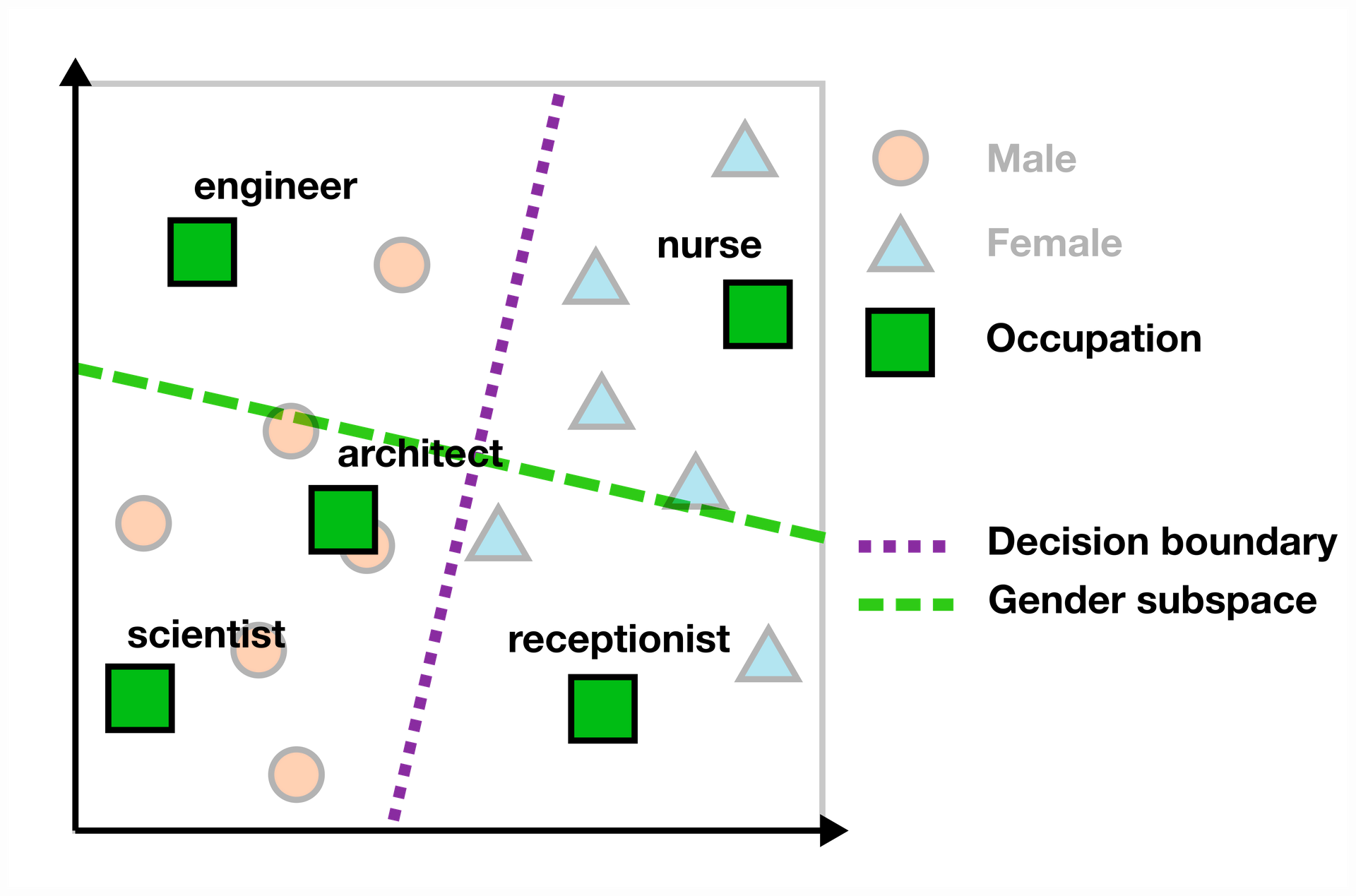Let us give an example of what we find if we apply this bias measure to the input embeddings of a Dutch LM called BERTje . When studying the gender bias for Dutch occupation terms, we find clear gender stereotypes in the top 5 male and female biased occupations, as can be seen in the table below.

Female bias (top 5) Male bias (top 5)
kinderopvang (child care) ingenieur (engineer)
verpleegkundige (nurse) chauffeur
verzorger (caretaker) kunstenaar (artist)